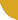|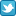Twitter |Facebook |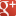Google+ |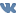VKontakte | LinkedIn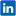|International Journal of Innovation and Scientific Research
ISSN: 2351-8014
 Saturday 30 May 2020

# Connect with IJISR

•••••••# ESSAI D'ELABORATION D'UNE THEORIE EXHAUSTIVE SUR LES EQUATIONS ALGEBRIQUES

Volume 13, Issue 2, February 2015, Pages 497–514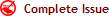Jean KYALULA USENI Bin KIBOFO

Original language: French

Copyright © 2015 ISSR Journals. This is an open access article distributed under the Creative Commons Attribution License, which permits unrestricted use, distribution, and reproduction in any medium, provided the original work is properly cited.

# Abstract

Mathematicians have tried to establish algorithms or formulas to solve the algebraic equations of the type of p(x) =0, where p(x) is a polynomial in x of degree n with real coefficients. That has been possible for n≤4. However, for n≥5. Abel proved that, in general, we cannot find formulas of resolution by radicals. Thus, mathematicians did not focus on the search of the resolubility condition any more by radicals of an algebraic equation of the n>5 degree. The present study discusses the theory of methods or the knowledge foundations on the algebraic equations. We also suggest the resolution of algebraic equation of n≤4 degree, and present the methods of resolution of the cells (equations) of the degree greater than or equal to 5 (n≥5) in the set of complex numbers.

Author Keywords: Algebraic, degree, equation, root, resolution.

# Abstract: (french)

Depuis toujours, les mathématiciens se sont attelés à établir des algorithmes ou formules permettant de résoudre des équations algébriques genre P(x)=0, ou P(x) est un polynôme en x de degré n à coefficient réels. Cela a pu être possible pour n≤4. Cependant, pour n≥5, le mathématicien Abel a prouvé qu'en général, on ne peut pas trouver des formules de résolution par radicaux. Ainsi, les mathématiciens n'ont plus focalisé leur attention sur la recherche de la condition de résolubilité par radicaux d'une équation algébrique de degré n≥5. La présente étude porte d'abord sur la théorie des méthodes ou des fondements de la connaissance sur les équations algébriques. Nous nous proposons ensuite d'organiser de façon raisonnée et complète la résolution des équations algébriques de degré n≤4 et de présenter les méthodes de résolution de celles de degré supérieur ou égal à 5 dans l'ensemble des nombres complexes.

Author Keywords: Algébrique, degré, équation, racine, résolution.